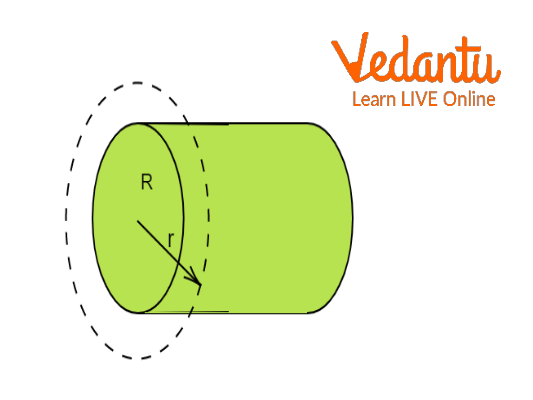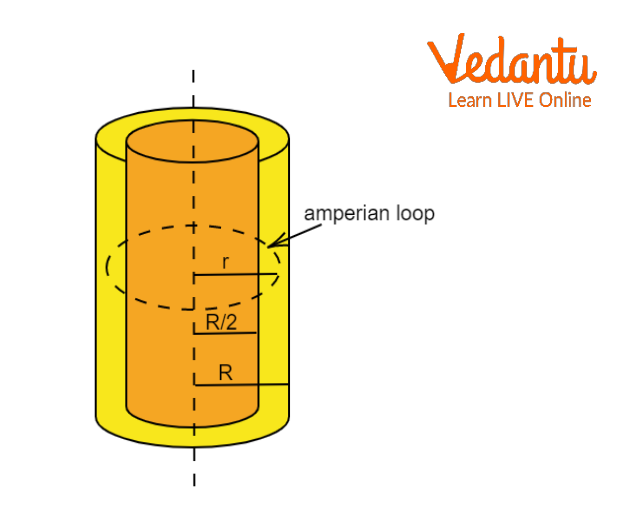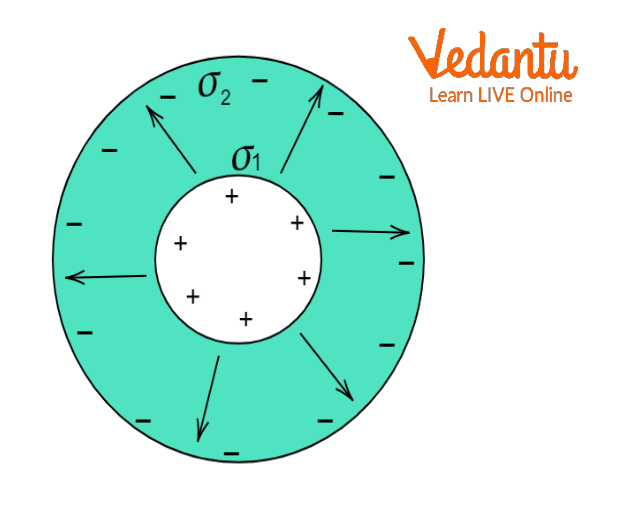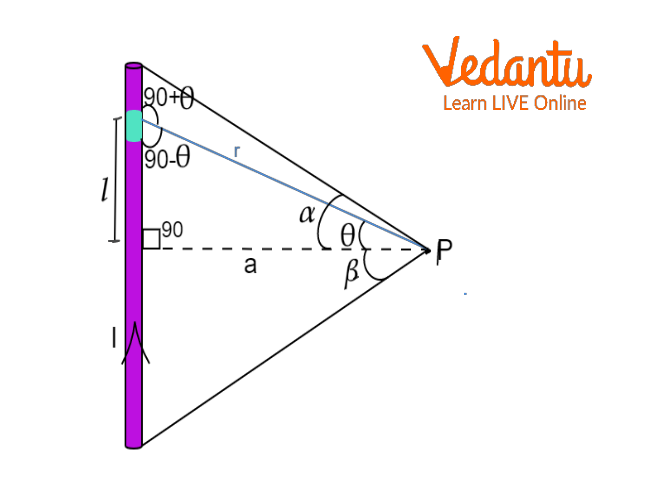Courses
Courses for Kids
Free study material
Free LIVE classes
More

# Magnetic Field Due to an Infinite Wire in Cylinder - JEE Important TopicLIVE
Join Vedantu’s FREE Mastercalss

## What are Magnetic Fields?

Moving electric charges generate magnetic fields. The flow of electric charges in different conductors give rise to a different varying magnetic field. An electric current through a metal wire will generate a differently varying magnetic field than a magnetic field generated when current is passed through a metal sheet. In this article, we shall talk about the magnetic field generated by an infinite current carrying cylindrical wire.

## Magnetic Field Due to a Cylindrical Wire

When an electric current is passed in a cylindrical wire, a magnetic field is generated. The generated magnetic field and its variation depends mainly on the type of wire i.e., solid or hollow and the region where we are measuring the magnetic field. The regions can be distinguished as follows:

• Outside the Cylinder

By using Ampere's law, we can calculate the magnetic field due to a cylindrical wire outside the cylindrical wire.

In all cases like in case of solid cylinder, thin hollow cylinder as well as thick hollow cylinder, we can write magnetic field at P as

$\oint{\vec{B}\cdot d\vec{l}}={{\mu }_{0}}i\Rightarrow B\int{dl=}{{\mu }_{0}}i$

$B\times 2\pi r={{\mu }_{0}}i\Rightarrow {{B}_{out}}=\frac{{{\mu }_{0}}i}{2\pi r}$

In all the above mentioned cases, BSurface $=\frac{{{\mu }_{0}}i}{2\pi R}$Magnetic Field Outside a Solid Cylinder

• Magnetic Field Inside A Hollow Cylinder

Here we will find what is the magnetic field inside a hollow cylindrical pipe. Magnetic field inside the hollow cylinder will be zero at any point. Also the electric field due to the hollow cylinder is zero.  This can be proved by Gauss law. Gauss law states that the total electric flux through a closed sphere is equal to the total charge enclosed inside the sphere divided by the electrical permittivity. So, if there is no electric field, then there is no active charge passing through a cylindrical conductor. And thus, there will be no magnetic field inside the hollow cylinder.

Let us take a point at a distance r from the centre of the hollow cylinder but inside the cylinder. According to the Gauss law,

${{\phi }_{r}}=\frac{{{Q}_{in}}}{{{\varepsilon }_{0}}}$

${{\phi }_{r}}=\frac{{{Q}_{in}}}{{{\varepsilon }_{0}}}$

$E\times 4\pi {{r}^{2}}=\frac{0}{{{\varepsilon }_{0}}}$

$E=0$Magnetic Field Inside the Hollow Cylinder

Thus , the magnetic field inside the hollow cylinder will be zero at any point.

• Inside the Solid Cylinder

If we are talking about the magnetic field at distance r from the axis where r<R then current enclosed in the region( i’) will be less than the total current i.

Current density is uniform so

$i'=i\times \frac{A'}{A}=i\frac{{{r}^{2}}}{{{R}^{2}}}$

Hence at point inside,$\oint{\vec{B}\cdot d\vec{l}}={{\mu }_{0}}i'$

$B=\frac{{{\mu }_{0}}ir}{2\pi {R^2}}$

• Inside the Thick Part of Hollow Cylinder

As current density is constant, current enclosed in a loop can be given as

${i}'=i\times \frac{{{A}'}}{A}$

$=i\frac{\left( {{r}^{2}}-R_{1}^{2} \right)}{\left( R_{2}^{2}-R_{1}^{2} \right)}$

So magnetic field at point Q is, $\oint{\vec{B}\cdot d\vec{l}}={{\mu }_{0}}i'$

$B=\frac{{{\mu }_{0}}i\left( {{r}^{2}}-R_{1}^{2} \right)}{2\pi \left( R_{2}^{2}-R_{1}^{2} \right)}$Magnetic Field Inside the Thick Part of Hollow Cylinder

### Magnetic Field for an Infinite Wire

Coming back to the main case, an infinitely long wire placed in space is carrying some current through it. As there is movement of electric charges, there shall be generation of a magnetic field. For an infinitely long wire, thickness is negligible compared to length. So, our main concern is calculating the magnetic field outside the wire. So, we can resolve it in the same way as the magnetic field outside the cylinder, which is  ${{B}_{out}}=\frac{{{\mu }_{0}}i}{2\pi r},$where r is distance from the axis.

So, we have the general formula for the magnetic field generated by an infinitely long wire.Magnetic Field for an Infinite Wire

Considering the nature, the magnetic field lines of an infinitely long wire are circular and centred at the wire, and they are identical in every plane perpendicular to the wire. Since the field decreases with distance from the wire, the spacing between field lines must decrease as well.

The direction of the magnetic field may be found by right hand thumb rule. If you hold the wire with your right hand so that your thumb points along the current then movement of fingers will travel in the same sense as the magnetic field vector. The direction of field lines can also be simulated by placing a compass needle near the wire. When current will be passed through the wire, the needles will align themselves tangent to the circle passing through them with the centre on the axis of the wire.

Same observation can be recorded by sprinkling iron filings on a horizontal surface. When the current is passed, iron filings will align themselves the same as compass needles.

## Conclusion

Magnetic field due to an infinite cylindrical wire is a special case of magnetic field generated by cylinders. As the length of the wire extends up to infinity, the intensity will only be dependent on the radial distance from the axis and obviously the current. We approached the concept that the line integral of the magnetic field around a closed path is equal to total current flowing through the area bounded by that closed path. We can apply this for other conductors as well to find the intensity of the magnetic field.

Last updated date: 02nd Oct 2023
Total views: 3.3k
Views today: 0.03k

## FAQs on Magnetic Field Due to an Infinite Wire in Cylinder - JEE Important Topic

1. Why is the behavior of the magnetic field the same for both solid and hollow cylinders when calculating outside the surface?

When we calculate the magnetic field outside the surface, the diameter of the wire is very small compared to the distance at which intensity is being calculated. Also, the effects of change of distance cancel each other out. So, it can be treated as a single wire placed at the axis and the variation of magnetic field is same for both the cases.

2. How does the magnetic field depend on the direction of the current?

The polarity of current decides the direction of flow of magnetic field as well. If the polarity of current is reversed then the direction of flow of magnetic field lines will also be revered. We can visualise the directions by using the second form of right-hand thumb rule. If you point your thumb in the direction of current flow, the travel of fingers when you will wrap them, will depict the direction of magnetic field lines. So, you will be able to see that when you reverse the direction of current then you need to point the thumb in the opposite direction as before, the movement of fingers will also be in the opposite direction. This is the change in direction of the magnetic field when current is reversed.